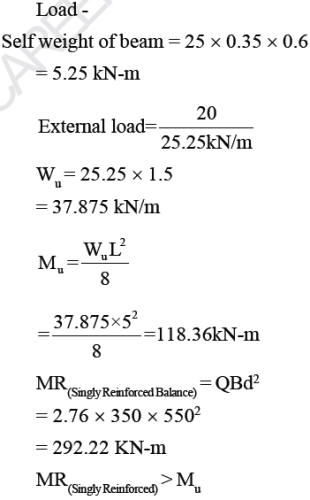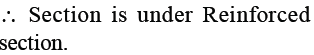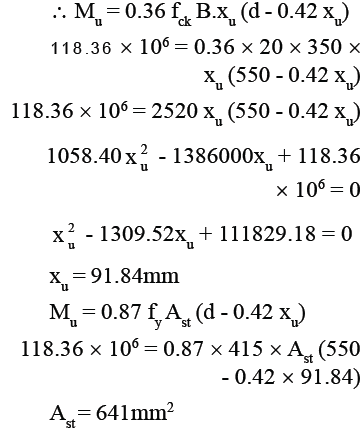Courses

# Test: RCC

## 25 Questions MCQ Test GATE Civil Engineering (CE) 2022 Mock Test Series | Test: RCC

Description
This mock test of Test: RCC for GATE helps you for every GATE entrance exam. This contains 25 Multiple Choice Questions for GATE Test: RCC (mcq) to study with solutions a complete question bank. The solved questions answers in this Test: RCC quiz give you a good mix of easy questions and tough questions. GATE students definitely take this Test: RCC exercise for a better result in the exam. You can find other Test: RCC extra questions, long questions & short questions for GATE on EduRev as well by searching above.
QUESTION: 1

### Assuming the concrete is uncracked, compute Bending stress in extreme fibres of Beam for Bending moment 5kN-m & axial load 200 kN use M25, Fe45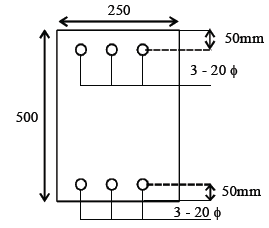Solution: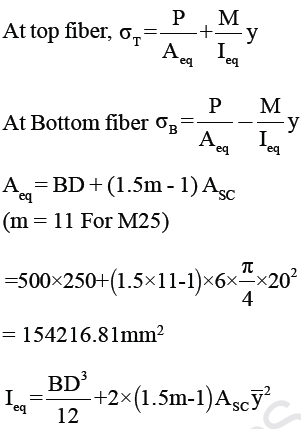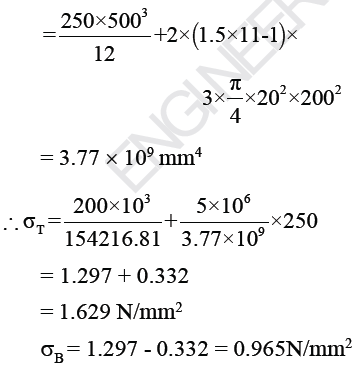QUESTION: 2

### In above question, determine cracking moment in kNm.

Solution:

For Cracking of section -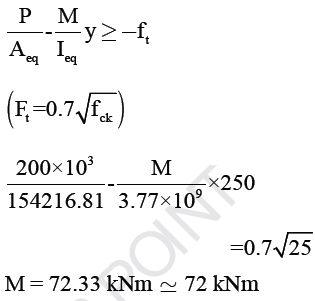QUESTION: 3

### Slab supported on wall on two opposite edges as given in figure. Thickness of wall is 250mm & thickness of slab is 175mm. Consider M20, Fe415 & effective cover 25mm. Find moment of Resistance of slab per meter width using LSM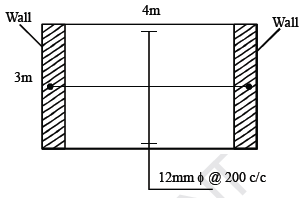Solution: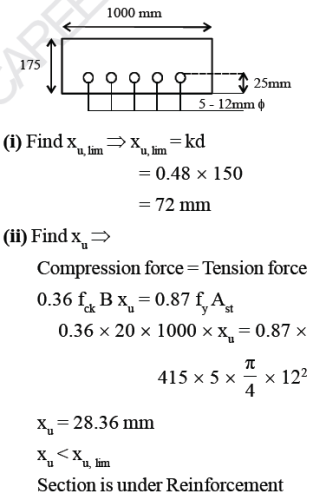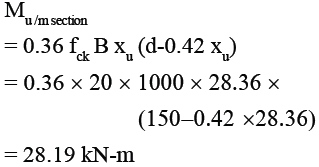QUESTION: 4

The maximum area of tension reinforcement in beams shall not exceed

Solution:

If tensile reinforcement of beam should exceed 4% of total gross area then some crack will be developed in concrete.

QUESTION: 5

A simply supported beam having effective length 8m subjected to a live load 6 kN/m the size of Beam is given below. Width of support is 300mm. Use M20/Fe415 use LSM. Find spacing for 2-legged 8mm φ - vertical stirrups. Use τc = 0.20 N/mm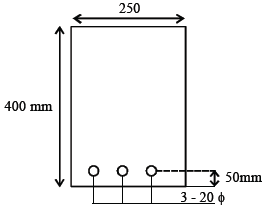Solution: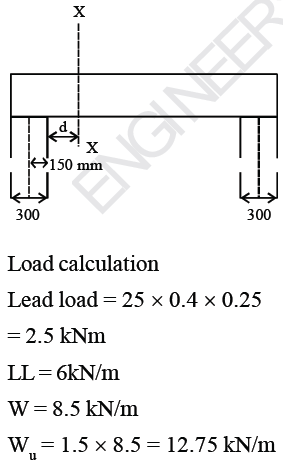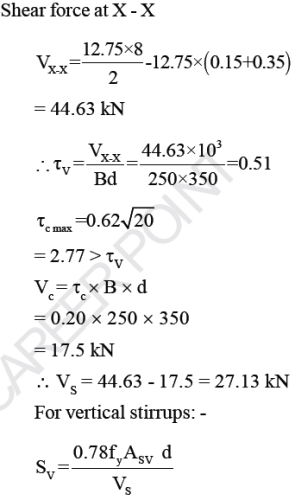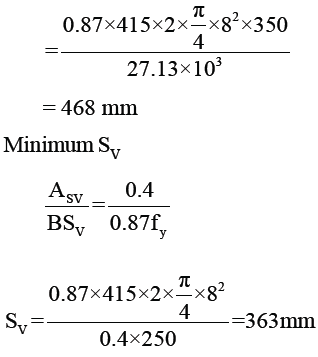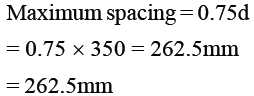*Answer can only contain numeric values
QUESTION: 6

In the previous question calculate bend stress. (in N/mm2)

(Important - Enter only the numerical value in the answer)

Solution: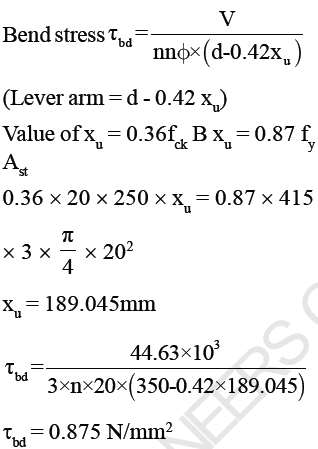QUESTION: 7

For a pretensioned beam, Young’s modulus of steel and concrete are 200 GPa and 35.35 GPa, respectively If the ultimate shrinkage strain and ultimate creep coefficient are 200 microns and 1.6 respectively, what is the level of sustained stress in concrete at the level of steel if the loss due to creep is three times the loss due to
shrinkage?

Solution: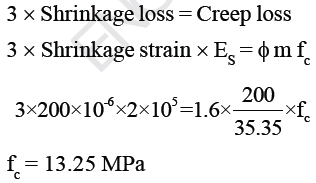QUESTION: 8

In a post tensioned pre-stressed concrete Beam. The end block zone is in between the end of the beam
and the section where

Solution:
QUESTION: 9

Find ultimate axial load carrying capacity of circular column having diameter 500mm. Consider clear cover 50mm. Column having 12Nos-20mm φ longitudinal Reinforcement & 8mm φ spiral Reinforcement.
Use M20/Fe415.

Solution: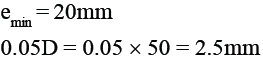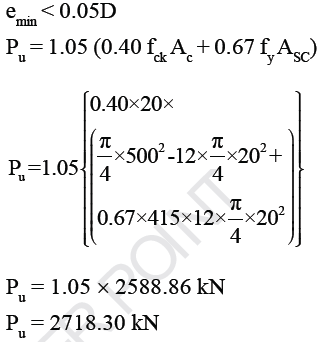QUESTION: 10

In above question find pitch of spiral Reinforcement

Solution: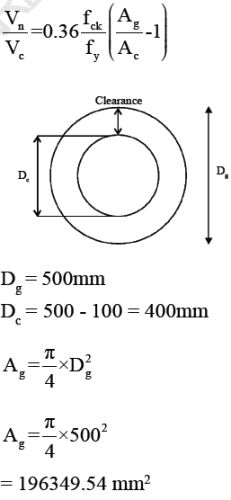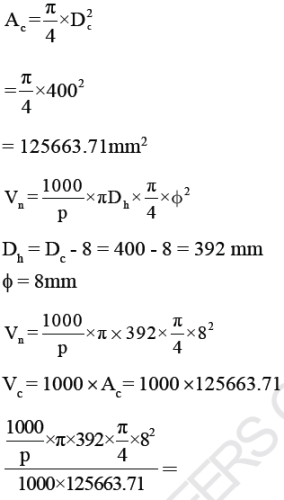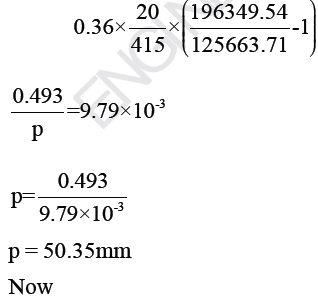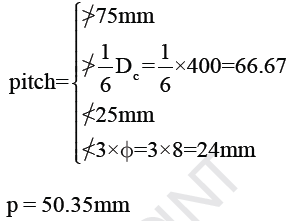QUESTION: 11

A simply supported post-tensioned prestressed concrete beam of span L is prestressed by a straight tendon
at a uniform eccentricity ‘e’ below the centroidal axis. If the magnitude of prestressing force is P and
flexural rigidity of beam is EI, maximum deflection of the beam is

Solution: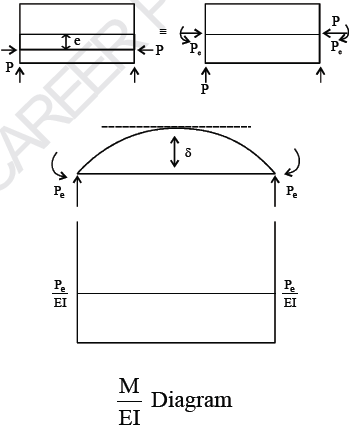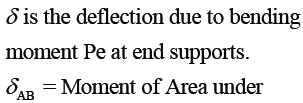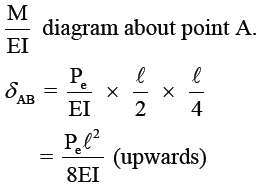QUESTION: 12

For maximum hogging bending moment at suppot in a continuous RC beam, live load should be placed on

Solution: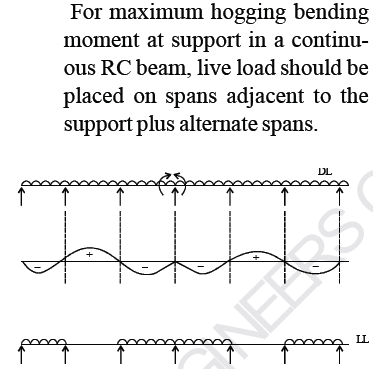QUESTION: 13

A box girder is subjected to loads as shown in the figure below. The critical section for shear in the bottom slab will occur at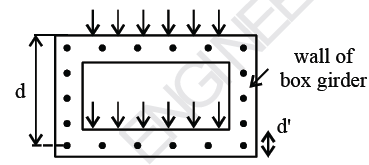Solution:

Since, the bottom slab will experience tension hence the critical section occurs at the face of wall.

QUESTION: 14

An isolated T-beam is used as a walkway. The beam is simply supported with an effective span of 6 m. The effective width of flange, for the cross-section shown in the figure is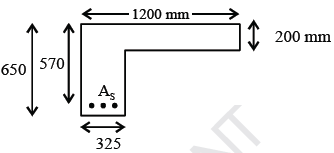Solution: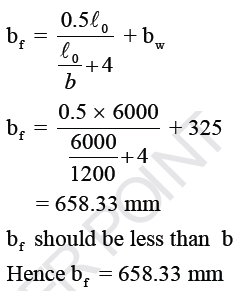QUESTION: 15

Shrinkage deflection in case of rectangular beams and slabs can be eliminated by putting

Solution:

Deflection due to shrinkage is caused due to differential shrinkage which is caused by
unsymmetrically placed reinforcement in beam. Hence, to avoid such deflection compression steel equal to tensile steel must be placed.

QUESTION: 16

The limits of percentage ‘p’ of the longitudinal reinforcement in a column is

Solution:

Minimum reinforcement in Column → 0.8%
Maximum reinforcement in Column → 6%

QUESTION: 17

A reinforced cantilever beam of span 7m, has a cross-sectional area of 250 mm X 500 mm (effective)
if checked for lateral stability and deflection, the beam will

Solution: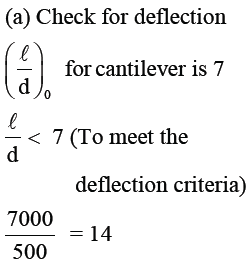Hence the beam will fail in
deflection

(b) Check for lateral stability :

For cantilever beam clear distance between the free end of cantilever and lateral restraint shall not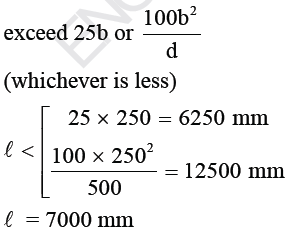Hence beam will fail in lateral stability.
Beam will fail in both deflection & lateral stability

QUESTION: 18

In an RCC beam of breadth ‘b’ and overall depth exceeding 750 mm, side face reinforcement required and allowable area of maximum tension reinforcement shall be respectively.

Solution:
*Answer can only contain numeric values
QUESTION: 19

Effective cover – 40 mm

Effective depth – 560 mm

Width of beam – 300 mm

Grade of Steel – Fe 415

Take m = 13, σcbc = 7 N/m2, σst = 230 N/mm2.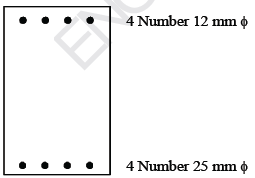Find moment of resistance of beam using working stress method __________

(Important - Enter only the numerical value in the answer)

Solution: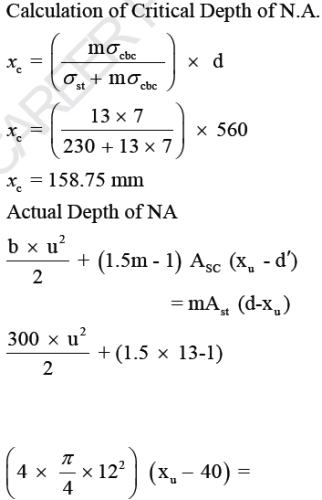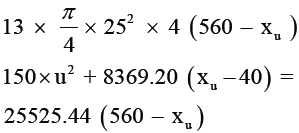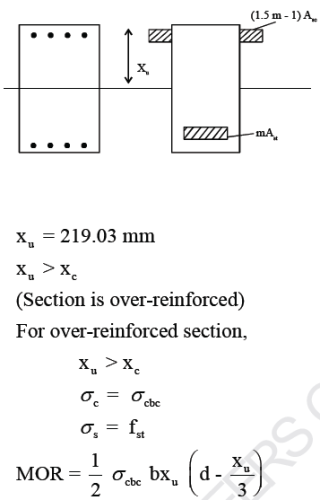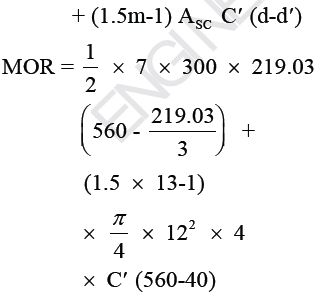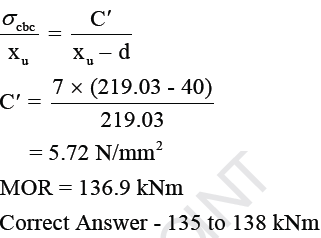QUESTION: 20

The equivalent bending moment for the beam having loading as follows is
BM = 300 kNm
SF = 120 kN
Torsional moment = 72 kNm
Use M 25/ Fe 415,

b = 400 mm,
d = 450 mm.

Effective cover = 50 mm

Solution: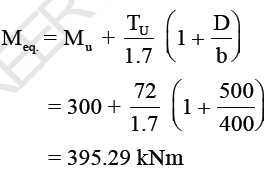QUESTION: 21

In the design of beams for unit limit state of collapse in flexure (as per IS : 456 : 2000), let the maximum strain in concrete be limited to 0.0025 (in place of 0.0035). For this situation, consider a rectangular beam section with breadth as 300 mm, effective depth as 450 mm, Area of tension steel as 1500 mm2, and characteristic
strength of concrete and steel as 30 Mpa and 250 Mpa respectively.

Q. The depth of neutral axis for the balanced failure is

Solution: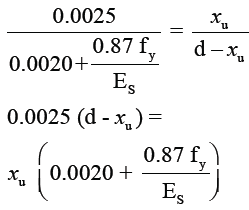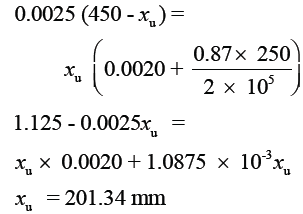QUESTION: 22

At the limiting state of collapse in flexure the force acting on the compression zone of the section is

Solution: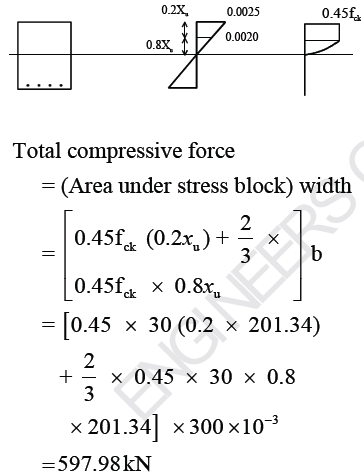QUESTION: 23

If characteristic compressive strength of concrete fck is defined as strength below which not more than 95% of the test result are expected to fall, the expression of fck in term of mean strength fm and standard deviation σ would be

Solution:
QUESTION: 24

The minimum and maximum percentage of tensile steel in following beam section is given as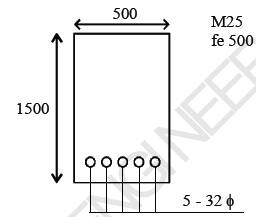Solution:

Minimum Ast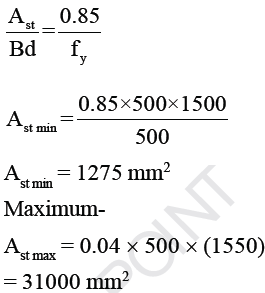QUESTION: 25

Find Area of tensile steel for following section. If required consider section as doubly
Reinforced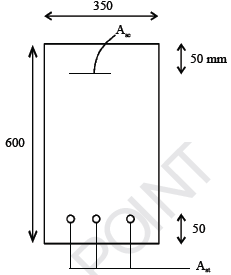If span of beam is 5m & external load is 20 kN/m. Use LSM. Beam is simply supported

Solution: